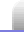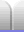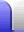# Number Sets

### Natural Numbers

The set of natural numbers are the numbers from 1 upward: 1, 2, 3, 4, 5, ...

Normally zero is not included in this set, because the natural numbers are the ones used naturally for counting. Hence, they are also called counting numbers.

The symbol for the natural numbers is blackboard bold N: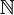.

### Whole Numbers

These are the natural numbers including zero: 0, 1, 2, 3, 4, 5, ...

### Integers

The set of integers include all natural numbers, zero, and negative versions of all of them as well: ..., -5, -4, -3, -2, -1, 0, 1, 2, 3, 4, 5, ...

The symbol for the integers set is blackboard bold Z: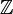, from the German word for numbers, zahlen.

### Rational Numbers

The set of rational numbers are any number that can be expressed as a fraction, so they are also simply called fractions. It thus includes the set of integers. When written as a decimal, some fractions are very easy to write because they terminate (their decimal places finish), while some never end, repeating one or more digits.

1/2 = 0.5

1/7 = 0.142857...

1/3 = 0.333...

2/3 = 0.666...

3/3 = 0.999...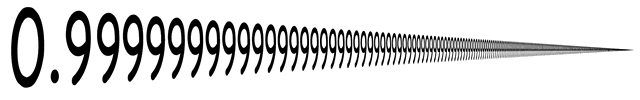∴ 0.999... ≡ 1

(The symbol means "therefore" and the symbol means "equivalent" or "is the same as", not just "has an equal value to".)

The symbol for the rational numbers is blackboard bold Q: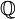, from quotient (the result of a division). For more on rational numbers see the Fractions Overview page.

### Irrational Numbers

These are numbers which, when written as a decimal, never end and never repeat. They cannot be expressed as fractions, so rational numbers and irrational numbers are separate sets.

Examples of irrational numbers include √2 and π.

There is no fixed symbol for irrational numbers. R \ Q (meaning the set of real numbers "set minus" the set of rational numbers) is the most common and the most likely to be understood.

### Real Numbers

The set of real numbers are all the rational numbers and irrational numbers together.

The symbol for the real numbers is blackboard bold R: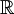.

### Complex Numbers

This set of numbers starts to get particularly interesting. Complex numbers have a real component and an imaginary component, so that a complex number is written as a + bi, where a and b are real numbers and i is the imaginary unit:

i2 = -1

See more about complex numbers on the Complex Numbers page.Home Astronomy Chemistry Electronics & Computers Mathematics Physics Field Trips Turn on javascript for email link